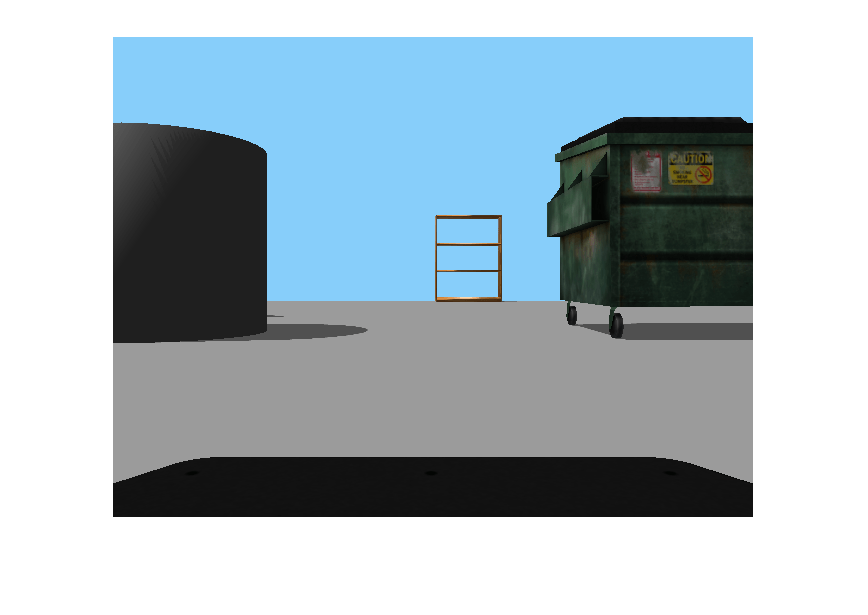Convert ROS image data into MATLAB image

## Syntax

``img = readImage(msg)``
``````[img,alpha] = readImage(msg)``````

## Description

example

````img = readImage(msg)` converts the raw image data in the message object, `msg`, into an image matrix, `img`. You can call `readImage` using either `'sensor_msgs/Image'` or `'sensor_msgs/CompressedImage'` messages.ROS image message data is stored in a format that is not compatible with further image processing in MATLAB®. Based on the specified encoding, this function converts the data into an appropriate MATLAB image and returns it in `img`.```
``````[img,alpha] = readImage(msg)``` returns the alpha channel of the image in `alpha`. If the image does not have an alpha channel, then `alpha` is empty.```

## Examples

collapse all

Load sample ROS messages including a ROS image message, `img`.

`exampleHelperROSLoadMessages`

Read the ROS image message as a MATLAB® image.

`image = readImage(img);`

Display the image.

`imshow(image)`## Input Arguments

collapse all

`'sensor_msgs/Image'` or `'sensor_msgs/CompressedImage'` ROS image message, specified as an `Image` or ```Compressed Image``` object handle.

## Output Arguments

collapse all

Image, returned as a matrix representing a grayscale or RGB image or as an m-by-n-by-3 array, depending on the sensor image.

Alpha channel, returned as a `uint8` grayscale image. If no alpha channel exists, `alpha` is empty.

Note

For `CompressedImage` messages, you cannot output an Alpha channel.

## Tips

ROS image messages can have different encodings. The encodings supported for images are different for `'sensor_msgs/Image'` and `'sensor_msgs/CompressedImage'` message types. Fewer compressed images are supported. The following encodings for raw images of size M-by-N are supported using the `'sensor_msgs/Image'` message type (`'sensor_msgs/CompressedImage'` support is in bold):

• `rgb8, rgba8, bgr8, bgra8`: `img` is an `rgb` image of size M-by-N-by-3. The alpha channel is returned in `alpha`. Each value in the outputs is represented as a `uint8`.

• `rgb16, rgba16, bgr16, and bgra16`: `img` is an RGB image of size M-by-N-by-3. The alpha channel is returned in `alpha`. Each value in the output is represented as a `uint16`.

• `mono8` images are returned as grayscale images of size M-by-N-by-1. Each pixel value is represented as a `uint8`.

• `mono16` images are returned as grayscale images of size M-by-N-by-1. Each pixel value is represented as a `uint16`.

• `32fcX` images are returned as floating-point images of size M-by-N-by-D, where D is 1, 2, 3, or 4. Each pixel value is represented as a `single`.

• `64fcX` images are returned as floating-point images of size M-by-N-by-D, where D is 1, 2, 3, or 4. Each pixel value is represented as a `double`.

• `8ucX` images are returned as matrices of size M-by-N-by-D, where D is 1, 2, 3, or 4. Each pixel value is represented as a `uint8`.

• `8scX` images are returned as matrices of size M-by-N-by-D, where D is 1, 2, 3, or 4. Each pixel value is represented as a `int8`.

• `16ucX` images are returned as matrices of size M-by-N-by-D, where D is 1, 2, 3, or 4. Each pixel value is represented as a `int16`.

• `16scX` images are returned as matrices of size M-by-N-by-D, where D is 1, 2, 3, or 4. Each pixel value is represented as a `int16`.

• `32scX` images are returned as matrices of size M-by-N-by-D, where D is 1, 2, 3, or 4. Each pixel value is represented as a `int32`.

• `bayer_X` images are returned as either Bayer matrices of size M-by-N-by-1, or as a converted image of size M-by-N-by-3 (Image Processing Toolbox™ is required).

The following encoding for raw images of size M-by-N is supported using the `'sensor_msgs/CompressedImage'` message type:

• `rgb8, rgba8, bgr8, and bgra8`: `img` is an `rgb` image of size M-by-N-by-3. The alpha channel is returned in `alpha`. Each output value is represented as a `uint8`.